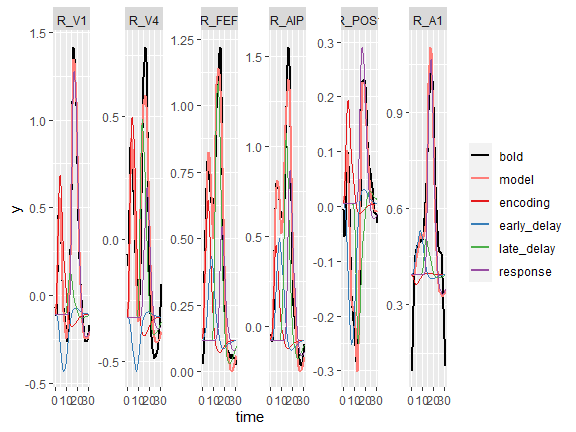# Analysis steps used in the spatial working memory study

#### 2022-11-19

Here, we present the analysis steps that were used for the evaluation of the autohrf package based on the spatial working memory study discussed in the paper Purg, N., Demšar, J., & Repovš, G. (2022). autohrf – an R package for generating data-informed event models for general linear modeling of task-based fMRI data. Frontiers in Neuroimaging.

We start the analysis by loading relevant libraries and the fMRI data collected during the spatial working memory study.

# libraries
library(tidyverse)
library(autohrf)

df <- swm
head(df)
##   roi t          y
## 1 L_1 0 0.02712162
## 2 L_1 1 0.06248649
## 3 L_1 2 0.12908108
## 4 L_1 3 0.30183784
## 5 L_1 4 0.51691892
## 6 L_1 5 0.65970270

The loaded data frame has 11520 observations, which correspond to the fMRI measurements for 360 different brain areas over 32 time points during a spatial working memory task trial. The fMRI data has been averaged for individual voxels within each region of interest (ROI), across individual task trials collected in one to three recording sessions per each participant, and across 37 participants. Each observation has three variables (roi, t, and y), where roi denotes the region of interest, t the time stamp and y the value of the BOLD signal.

To visualize the data we select six ROIs with different types of activity during the spatial working memory task and plot their mean activity during a task trial.

# prepare relevant ROIs for visualization
roisv <- c("R_V1", "R_V4", "R_FEF", "R_AIP", "R_POS1", "R_A1")

# view data of selected ROIs
df %>%
filter(roi %in% roisv) %>%
mutate(roi = factor(roi, levels=roisv)) %>%
ggplot(aes(t, y)) +
geom_line(size=0.8) +
facet_wrap(~ roi, nrow = 1)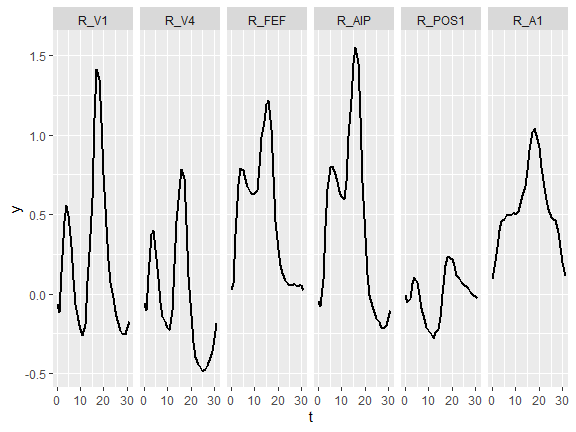Next, we evaluate and compare different hypothesized models with a varying number of event predictors. Specifically, we prepare four models, which are manually evaluated based on the activity across 360 ROIs measured during the spatial working memory study. Finally, we visualize model fits to the BOLD signal based on six example ROIs that show different types of activity during a spatial working memory task.

# prepare models
model1 <- data.frame(event = c("encoding", "delay", "response"),
start_time = c(0, 0.15, 10),
duration = c(0.15, 9.85, 3))

model2 <- data.frame(event = c("encoding", "early_delay", "late_delay", "response"),
start_time = c(0, 0.15, 5, 10),
duration = c(0.15, 4.85, 5, 3))

model3 <- data.frame(event = c("encoding", "delay", "probe", "response"),
start_time = c(0, 0.15, 10, 10.5),
duration = c(0.15, 9.85, 0.5, 2.5))

model4 <- data.frame(event = c("stimulus", "encoding", "delay", "probe", "response"),
start_time = c(0, 0.15, 2, 10, 10.5),
duration = c(0.15, 1.85, 8, 0.5, 2.5))

# evaluate models
em1 <- evaluate_model(df, model1, tr = 1, hrf = "spm")
##
## Mean R2:  0.6730635
## Median R2:  0.7901452
## Min R2:  0.008685168
## Weighted R2:  0.6730635
##
## Mean BIC:  -40.86529
## Median BIC:  -40.42351
## Min BIC:  -115.1637
## Weighted BIC:  -40.86529
em2 <- evaluate_model(df, model2, tr = 1, hrf = "spm")
##
## Mean R2:  0.7328098
## Median R2:  0.8205526
## Min R2:  0.1015643
## Weighted R2:  0.7328098
##
## Mean BIC:  -44.62028
## Median BIC:  -44.93817
## Min BIC:  -117.9587
## Weighted BIC:  -44.62028
em3 <- evaluate_model(df, model3, tr = 1, hrf = "spm")
##
## Mean R2:  0.8149324
## Median R2:  0.8599957
## Min R2:  0.1459216
## Weighted R2:  0.8149324
##
## Mean BIC:  -53.81372
## Median BIC:  -53.69716
## Min BIC:  -142.6516
## Weighted BIC:  -53.81372
em4 <- evaluate_model(df, model4, tr = 1, hrf = "spm")
##
## Mean R2:  0.8252129
## Median R2:  0.874879
## Min R2:  0.170891
## Weighted R2:  0.8252129
##
## Mean BIC:  -53.60671
## Median BIC:  -53.55636
## Min BIC:  -141.3314
## Weighted BIC:  -53.60671
# plot model fits
plot_model(em1, by_roi = TRUE, rois = roisv, nrow = 1)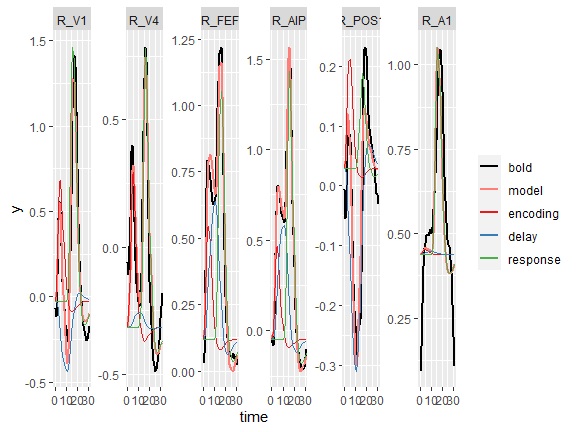plot_model(em2, by_roi = TRUE, rois = roisv, nrow = 1)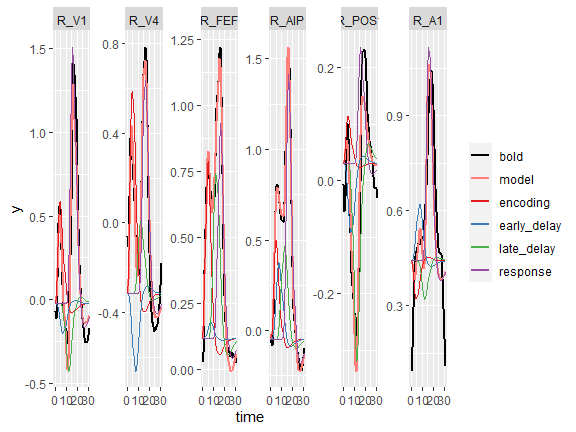plot_model(em3, by_roi = TRUE, rois = roisv, nrow = 1)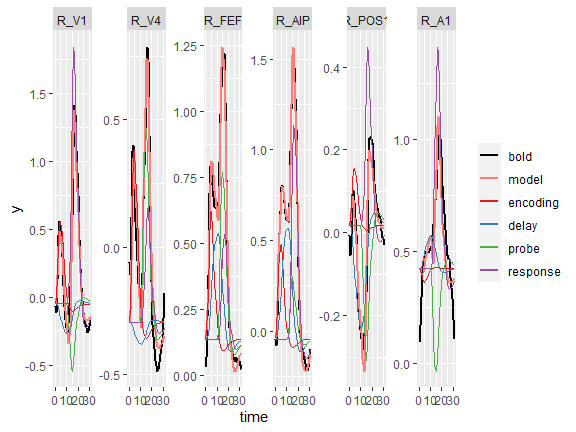plot_model(em4, by_roi = TRUE, rois = roisv, nrow = 1)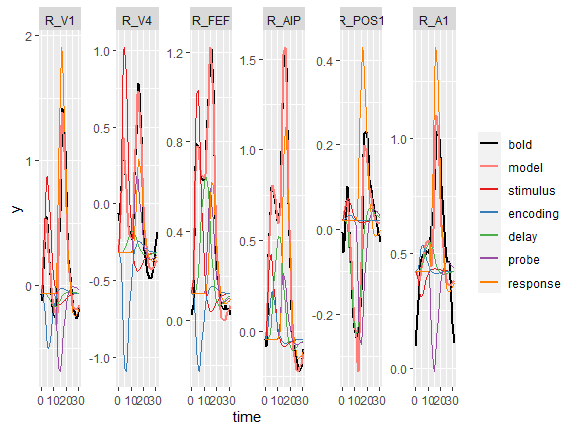We continue with the comparison of theoretical and data-driven models obtained with autohrf. For that purpose, we extract 80 ROIs within brain systems that are commonly associated with spatial working memory.

# prepare relevant ROIs for autohrf
roisa <- c("R_V1", "R_V2", "R_V3", "R_V4", "R_IPS1", "R_4", "R_3a", "R_3b",
"R_1", "R_2", "R_6mp", "R_6ma", "R_SCEF", "R_6d", "R_6a", "R_FEF",
"R_6v", "R_6r", "R_PEF", "R_LIPv", "R_LIPd", "R_VIP", "R_AIP",
"R_MIP", "R_7PC", "R_7AL", "R_7Am", "R_7PL", "R_7Pm", "R_PFm",
"R_PF", "R_PFt", "R_PFop", "R_IP0", "R_IP1", "R_IP2", "R_p9-46v",
"R_a9-46v", "R_46", "R_9-46d", "L_V1", "L_V2", "L_V3", "L_V4",
"L_IPS1", "L_4", "L_3a", "L_3b", "L_1", "L_2", "L_6mp", "L_6ma",
"L_SCEF", "L_6d", "L_6a", "L_FEF", "L_6v", "L_6r", "L_PEF",
"L_LIPv", "L_LIPd", "L_VIP", "L_AIP", "L_MIP", "L_7PC", "L_7AL",
"L_7Am", "L_7PL", "L_7Pm", "L_PFm", "L_PF", "L_PFt", "L_PFop",
"L_IP0", "L_IP1", "L_IP2", "L_p9-46v",
"L_a9-46v", "L_46", "L_9-46d")

# extract data for autohrf
dfa <- df %>%
filter(roi %in% roisa)

We run automated parameter search using autohrf based on two models, one with three event predictors and the other with four event predictors. For each model, we evaluate one theoretically assumed model and prepare two automatically derived models based on the actual fMRI data, one with strict constraints and another with permissive constraints.

# Model 1 with events encoding, delay, and response

# prepare model constraints
modelA <- data.frame(event = c("encoding", "delay", "response"),
start_time = c(0, 0.15, 10),
end_time = c(0.15, 10, 13),
min_duration = c(0.15, 9.85, 3),
max_duration = c(0.15, 9.85, 3))

modelB <- data.frame(event = c("encoding", "delay", "response"),
start_time = c(0, 0.15, 10),
end_time = c(0.15, 10, 13),
min_duration = c(0.05, 5, 1.5),
max_duration = c(0.15, 9.85, 3))

modelC <- data.frame(event = c("encoding", "delay", "response"),
start_time = c(0, 0, 9),
end_time = c(1, 11, 14),
min_duration = c(0.05, 5, 1.5),
max_duration = c(1, 11, 5))

# join models
models <- list(modelA, modelB, modelC)

# run autohrf (to speed vignette building we load results from a previous autohrf run)
# autofit1 <- autohrf(dfa, models, tr = 1, iter = 500, allow_overlap = TRUE)
autofit1 <- swm_autofit1

# plot models' fitness
plot_fitness(autofit1) +
scale_color_brewer(labels=c("theoretical", "strict", "permissive"), type = "qual", palette = "Set1")
## Scale for 'colour' is already present. Adding another scale for 'colour',
## which will replace the existing scale.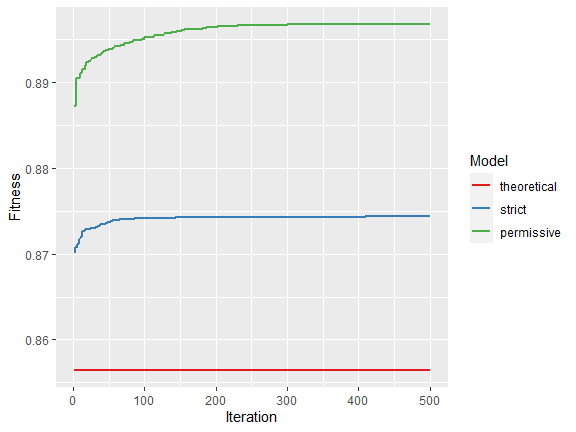# plot regressors
plot_best_models(autofit1)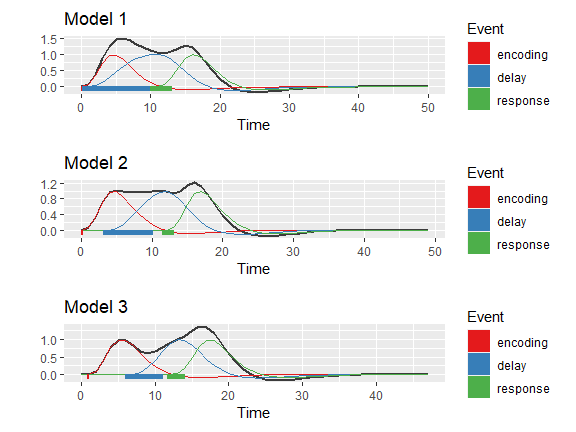# return derived parameters
best_models <- get_best_models(autofit1)
##
## ----------------------------------------
##
## Model 1
##
## Fitness:  0.8564832
##
##      event start_time duration
## 1 encoding       0.00     0.15
## 2    delay       0.15     9.85
## 3 response      10.00     3.00
##
## ----------------------------------------
##
## ----------------------------------------
##
## Model 2
##
## Fitness:  0.8743495
##
##      event  start_time   duration
## 1 encoding  0.07616286 0.07110048
## 2    delay  3.14017919 6.85982081
## 3 response 11.50000000 1.50000000
##
## ----------------------------------------
##
## ----------------------------------------
##
## Model 3
##
## Fitness:  0.8967331
##
##      event start_time  duration
## 1 encoding  0.8950581 0.1046247
## 2    delay  6.0000000 5.0000000
## 3 response 11.6009077 2.3990923
##
## ----------------------------------------
# evaluate models
modelA <- best_models[]
emA <- evaluate_model(df, modelA, tr = 1, hrf = "spm")
##
## Mean R2:  0.6730635
## Median R2:  0.7901452
## Min R2:  0.008685168
## Weighted R2:  0.6730635
##
## Mean BIC:  -40.86529
## Median BIC:  -40.42351
## Min BIC:  -115.1637
## Weighted BIC:  -40.86529
plot_model(emA, by_roi = TRUE, rois = roisv, nrow = 1)modelB <- best_models[]
emB <- evaluate_model(df, modelB, tr = 1, hrf = "spm")
##
## Mean R2:  0.6956795
## Median R2:  0.7761656
## Min R2:  0.01318867
## Weighted R2:  0.6956795
##
## Mean BIC:  -39.70276
## Median BIC:  -39.08748
## Min BIC:  -111.9774
## Weighted BIC:  -39.70276
plot_model(emB, by_roi = TRUE, rois = roisv, nrow = 1)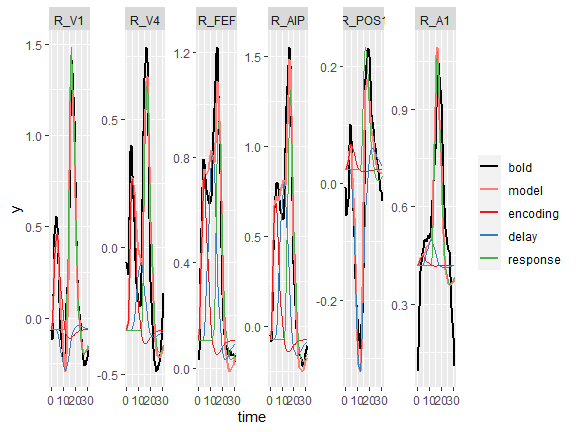modelC <- best_models[]
emC <- evaluate_model(df, modelC, tr = 1, hrf = "spm")
##
## Mean R2:  0.7437816
## Median R2:  0.8203383
## Min R2:  0.06211361
## Weighted R2:  0.7437816
##
## Mean BIC:  -45.47706
## Median BIC:  -46.56427
## Min BIC:  -128.2322
## Weighted BIC:  -45.47706
plot_model(emC, by_roi = TRUE, rois = roisv, nrow = 1)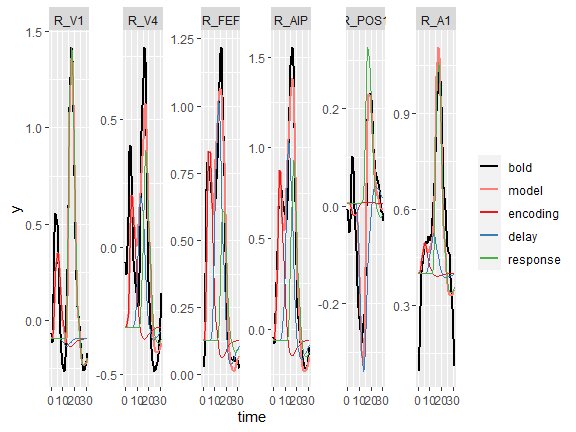# Model 2 with events encoding, early delay, late delay & response

# prepare models
modelA <- data.frame(event = c("encoding", "early_delay", "late_delay", "response"),
start_time = c(0, 0.15, 5, 10),
end_time = c(0.15, 5, 10, 13),
min_duration = c(0.15, 4.85, 5, 3),
max_duration = c(0.15, 4.85, 5, 3))

modelB <- data.frame(event = c("encoding", "early_delay", "late_delay", "response"),
start_time = c(0, 0.15, 5, 10),
end_time = c(0.15, 5, 10, 13),
min_duration = c(0.05, 2.5, 2.5, 1.5),
max_duration = c(0.15, 4.85, 5, 3))

modelC <- data.frame(event = c("encoding", "early_delay", "late_delay", "response"),
start_time = c(0, 0, 4, 9),
end_time = c(1, 6, 11, 14),
min_duration = c(0.05, 2.5, 2.5, 1.5),
max_duration = c(1, 6, 6, 5))

# join models
models <- list(modelA, modelB, modelC)

# run autohrf (to speed vignette building we load results from a previous autohrf run)
# autofit2 <- autohrf(dfa, models, tr = 1, iter = 200, allow_overlap = TRUE)
autofit2 <- swm_autofit2

# plot models" fitness
plot_fitness(autofit2) +
scale_color_brewer(labels=c("theoretical", "strict", "permissive"), type = "qual", palette = "Set1")
## Scale for 'colour' is already present. Adding another scale for 'colour',
## which will replace the existing scale.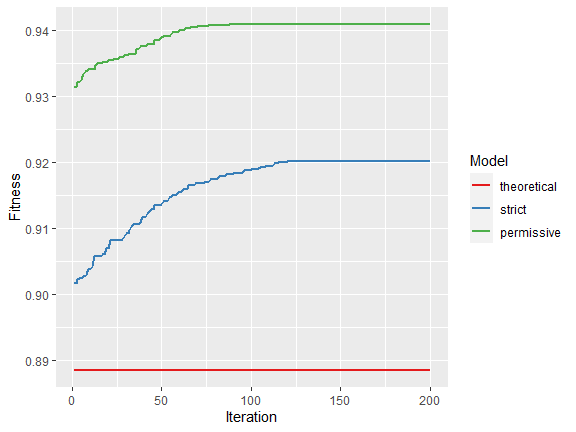# plot regressors
plot_best_models(autofit2)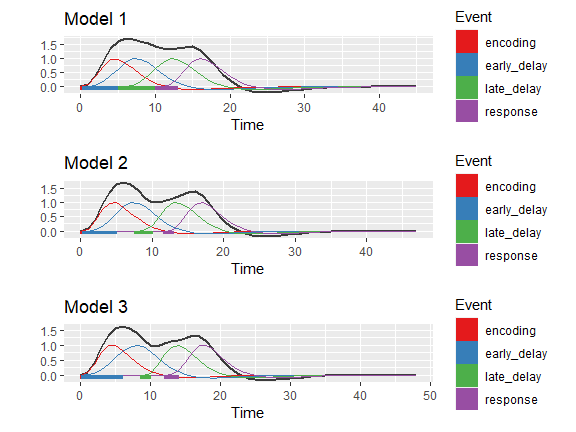# return derived parameters
best_models <- get_best_models(autofit2)
##
## ----------------------------------------
##
## Model 1
##
## Fitness:  0.8885289
##
##         event start_time duration
## 1    encoding       0.00     0.15
## 2 early_delay       0.15     4.85
## 3  late_delay       5.00     5.00
## 4    response      10.00     3.00
##
## ----------------------------------------
##
## ----------------------------------------
##
## Model 2
##
## Fitness:  0.9202106
##
##         event   start_time   duration
## 1    encoding  0.004645893 0.08965006
## 2 early_delay  0.150000000 4.85000000
## 3  late_delay  7.500000000 2.50000000
## 4    response 11.500000000 1.50000000
##
## ----------------------------------------
##
## ----------------------------------------
##
## Model 3
##
## Fitness:  0.94093
##
##         event   start_time   duration
## 1    encoding  0.003531708 0.07125868
## 2 early_delay  0.030498808 5.95715262
## 3  late_delay  8.500000000 1.50000000
## 4    response 11.915922371 2.08407763
##
## ----------------------------------------
# evaluate models
modelA <- best_models[]
emA <- evaluate_model(df, modelA, tr = 1, hrf = "spm")
##
## Mean R2:  0.7328098
## Median R2:  0.8205526
## Min R2:  0.1015643
## Weighted R2:  0.7328098
##
## Mean BIC:  -44.62028
## Median BIC:  -44.93817
## Min BIC:  -117.9587
## Weighted BIC:  -44.62028
plot_model(emA, by_roi = TRUE, rois = roisv, nrow = 1)modelB <- best_models[]
emB <- evaluate_model(df, modelB, tr = 1, hrf = "spm")
##
## Mean R2:  0.783997
## Median R2:  0.8566813
## Min R2:  0.1337443
## Weighted R2:  0.783997
##
## Mean BIC:  -50.46491
## Median BIC:  -51.52933
## Min BIC:  -129.965
## Weighted BIC:  -50.46491
plot_model(emB, by_roi = TRUE, rois = roisv, nrow = 1)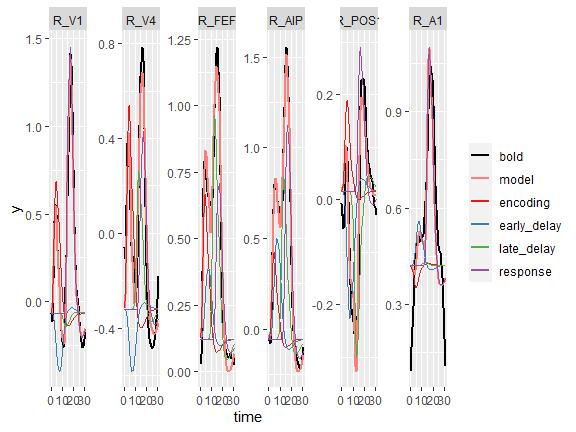modelC <- best_models[]
emC <- evaluate_model(df, modelC, tr = 1, hrf = "spm")
##
## Mean R2:  0.8243079
## Median R2:  0.8763235
## Min R2:  0.1705178
## Weighted R2:  0.8243079
##
## Mean BIC:  -55.63547
## Median BIC:  -56.1995
## Min BIC:  -138.4166
## Weighted BIC:  -55.63547
plot_model(emC, by_roi = TRUE, rois = roisv, nrow = 1)